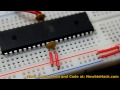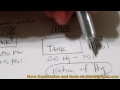### Flex Sensor - Senses Bending and DisplacementIntroduction:

Measure bending and flex with this sensor. The more this sensor is bent, the more resistance will be across the leads. 55.37 mm (2.18 inches) in length.

Description:

In its flat position, you can expect 25K ohms of resistance. All resistances will have a 30% tolerance. According to the datasheet, bending the sensor, you can expect a max of 125K ohms of resistance. Check below what my own tests yielded.

You will be able to bend and flex this sensor more than one millions times. The flex sensor can handle a continuous power of .50 watts, but don't exceed 1 watt.

This flex sensor can be applied in so many ways, like robotics, medical devices, gaming haptic feedback, user interface devices for computers, musical instruments, physical therapy, or many other uses. Imagine using this in a glove to sense the fingers being bent or using it to vary pitch in a sound. The possibilities are endless.

To apply this in a scenario for microcontrollers, you will want to use it with the ADC (analog to digital converter). You will need to use this in a voltage dividing circuit.

You can see the voltage divider circuit in the image. Applying this voltage divider, you will notice that R2 cannot be modified to product a full range of voltage from, say 0v to 5v. So you will need to pick a value that will give you the most range for Vout as possible. In my tests, R1, the flex sensor, gave me about 24k to 25k in its straight position and about 70k in its bent position. Now you can apply this to the voltge divider formula. I make quick work to find the widest possible range simply by using Excel and plugged in the voltage divider formula. I found that 42k gave me the best range showing a difference of 1.259. At the 25k (flat position), the Vout would be 3.13V and at 70k (fully bent), the Vout would be 1.875V. At 10-bits of ADC, that would give you a fair amount to play with.

So, what if you didn't need the flex sensor to bend that much. Say, you wanted only a slight bend. Let's make the bend range from 25k to 40k. You would want to select a 32k resistor for R2 which would give you a voltage range of .5847

55.37mm (2.18 Inch) Flex Sensor

\$8.50
Qty:

### Learn about the Flex Sensor - Senses Bending and DisplacementTo have the microcontroller read a pressure sensor, the sensor is connected to the ADC (Analog to Digital Converter). In this case, the sensor is connected to the pin 0 of the ADC (PORT A). AVCC (...When the pressure sensor is tested to see the raw ADC 10-bit data on the LCD, the actual data did not appear. In the program, the bitwise operation for shifting and setting the ADEN (ADC Enable) to 1...The LCD shows the results of a raw output from the ADC reading the pressure sensor. A simple test of the pressure sensor was made successfully.This is a great vacuum pressure sensor that can sense up to about 40 inches of mercury (Hg) which is -115 KPa (Kilopascals). This range is perfect for many industrial uses for vacuum, including pick a...The LCD library and initialization is established. The interrupts for the ADC is started and a string is sent to the LCD. This is to get ready for the pressure sensor program. A string is sent to t...